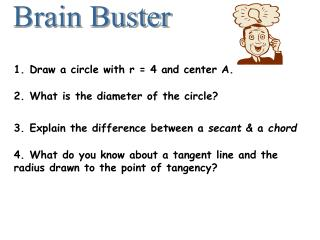DownloadDownload PresentationBrain Buster

# Brain Buster

Download Presentation## Brain Buster

- - - - - - - - - - - - - - - - - - - - - - - - - - - E N D - - - - - - - - - - - - - - - - - - - - - - - - - - -
##### Presentation Transcript

1. Brain Buster 1. Draw a circle with r = 4 and center A. 2. What is the diameter of the circle? 3. Explain the difference between a secant & a chord 4. What do you know about a tangent line and the radius drawn to the point of tangency?

2. Math II UNIT QUESTION: What special properties are found with the parts of a circle? Standard: MM2G1, MM2G2 Today’s Question: How do we use angle measures to find measures of arcs? Standard: MM2G3.a,d

3. Arcs and Section 6.2, 6.3 Chords

4. Central Angle : An Angle whose vertex is at the center of the circle ACB AB A Major Arc Minor Arc More than 180° Less than 180° P To name: use 3 letters C To name: use 2 letters B APB is a Central Angle

5. EDF Semicircle: An Arc that equals 180° To name: use 3 letters E D P F

6. THINGS TO KNOW AND REMEMBER ALWAYS A circle has 360 degrees A semicircle has 180 degrees Vertical Angles are Equal

7. measure of an arc = measure of central angle m AB m ACB m AE A E 96 Q = 96° B C = 264° = 84°

8. Arc Addition Postulate m ABC = m AB + m BC A C B

9. m DAB = Tell me the measure of the following arcs. 240 D A 140 260 m BCA = R 40 100 80 C B

10. CONGRUENT ARCS Congruent Arcs have the same measure and MUST come from the same circle or of congruent circles. C B D 45 45 110 A

11. In the same circle, or in congruent circles, two minor arcs are congruent if and only if their corresponding chords are congruent. B C AB  CD IFF AB  DC A D

12. Ex. 1 60 120 120 x x = 60

13. Ex. 2 2x x + 40 2x = x + 40 x = 40

14. What can you tell me about segment AC if you know it is the perpendicular bisectors of segments DB? D It’s the DIAMETER!!! A C B

15. Ex. 3 If a diameter of a circle is perpendicular to a chord, then the diameter bisects the chord and its arc. x = 24 24 y 60 y = 30 x

16. Example 4 EX 2: In P, if PM  AT, PT = 10, and PM = 8, find AT. P A M MT = 6 T AT = 12

17. Example 5 In R, XY = 30, RX = 17, and RZ  XY. Find RZ. X RZ = 8 R Z Y

18. Example 6 IN Q, KL  LZ. IF CK = 2X + 3 and CZ = 4x, find x. Q x = 1.5 C Z K L

19. In the same circle or in congruent circles, two chords are congruent if and only if they are equidistant from the center. B AD  BC IFF LP  PM A M P L C D

20. Ex. 7: InA, PR = 2x + 5 and QR = 3x –27. Find x. R A x = 32 Q P

21. Ex. 8: IN K, K is the midpoint of RE. If TY = -3x + 56 and US = 4x, find x. U T K E R S x = 8 Y